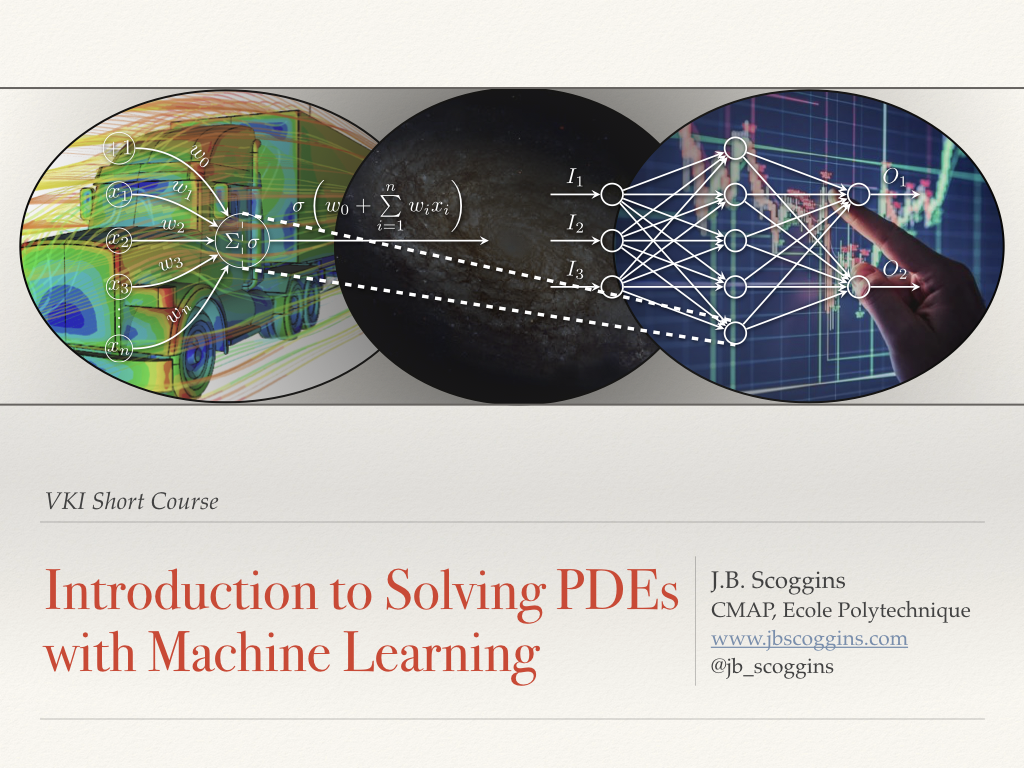I gave a short course on Solving PDEs with Machine Learning at VKI on Jan. 27. All the material for the short course can be found on my git.Abstract

Solving partial differential equations is an engineer’s bread and butter. Classical discretization techniques such as finite-difference or finite-volume are powerful methods for the numerical solution of PDEs, however, their reliance on robust and efficient mesh generation often presents difficulties in solution convergence or necessitates enormous computational resources to obtain an accurate solution. In this lecture, an alternative method based on a statistical machine learning approach will be presented, which yields a continuous, differentiable, and meshless representation of the hidden PDE solution. The topics covered will include machine learning basics, foundations in neural networks, and PDE solution techniques with neural networks. Following the lecture, a practical session on solving PDEs will be presented using the Tensorflow library and Jupyter notebooks with Google Colab. All lecture slides and Jupyter notebooks will be available after the lecture.